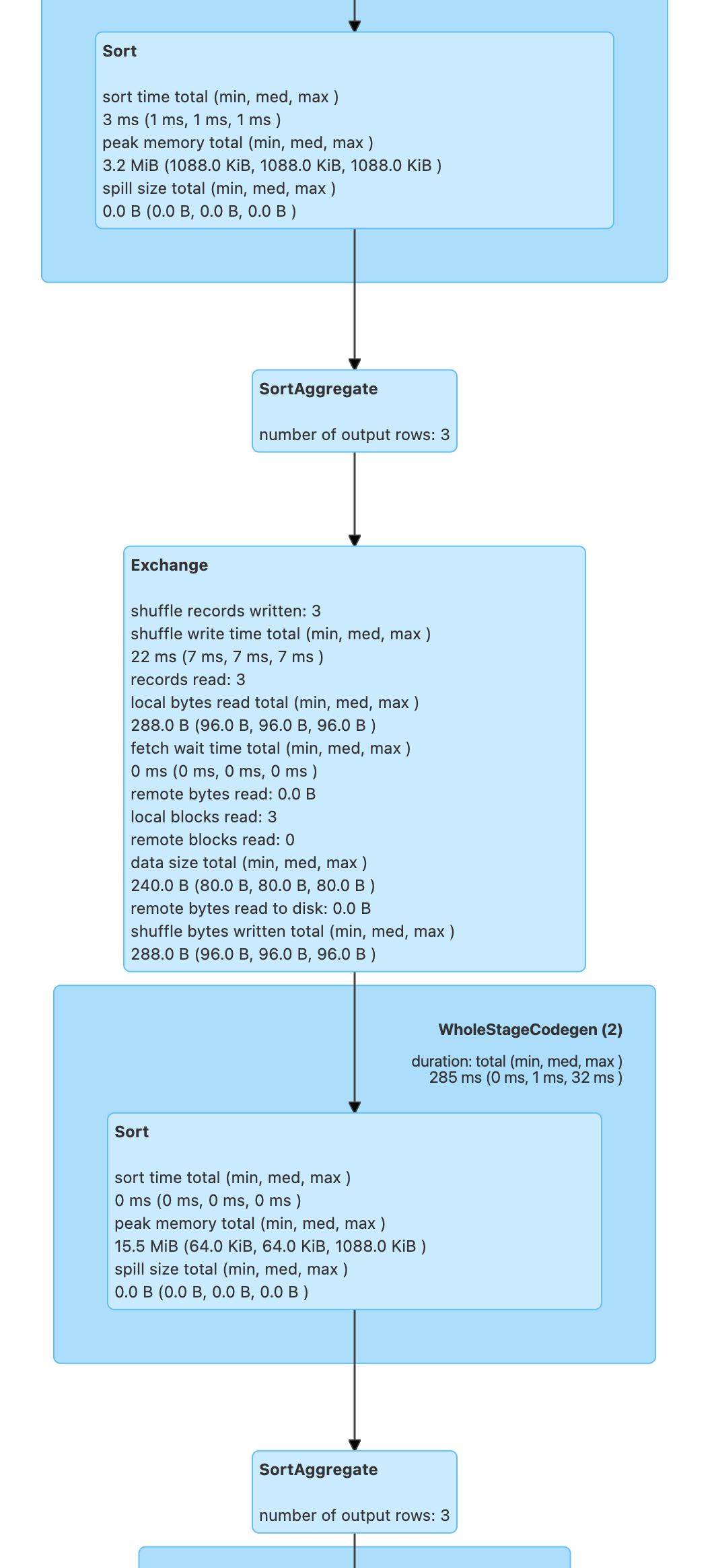# SortAggregateExec Aggregate Physical Operator¶

`SortAggregateExec` is an aggregate unary physical operator for sort-based aggregation.## Creating Instance¶

`SortAggregateExec` takes the following to be created:

`SortAggregateExec` is created when:

## Performance Metrics¶

Key Name (in web UI)
numOutputRows number of output rows

## Demo¶

Let's disable preference for ObjectHashAggregateExec physical operator (using the spark.sql.execution.useObjectHashAggregateExec configuration property).

``````spark.conf.set("spark.sql.execution.useObjectHashAggregateExec", false)
assert(spark.sessionState.conf.useObjectHashAggregation == false)
``````
``````val names = Seq(
(0, "zero"),
(1, "one"),
(2, "two")).toDF("num", "name")
``````

Let's use immutable data types for `aggregateBufferAttributes` (so HashAggregateExec physical operator will not be selected).

``````val q = names
.withColumn("initial", substring('name, 0, 1))
.groupBy('initial)
.agg(collect_set('initial))
``````
``````scala> q.explain
== Physical Plan ==
SortAggregate(key=[initial#160], functions=[collect_set(initial#160, 0, 0)])
+- *(2) Sort [initial#160 ASC NULLS FIRST], false, 0
+- Exchange hashpartitioning(initial#160, 200), ENSURE_REQUIREMENTS, [id=#122]
+- SortAggregate(key=[initial#160], functions=[partial_collect_set(initial#160, 0, 0)])
+- *(1) Sort [initial#160 ASC NULLS FIRST], false, 0
+- *(1) LocalTableScan [initial#160]
``````
``````import q.queryExecution.optimizedPlan
import org.apache.spark.sql.catalyst.plans.logical.Aggregate
val aggLog = optimizedPlan.asInstanceOf[Aggregate]
import org.apache.spark.sql.catalyst.planning.PhysicalAggregation
import org.apache.spark.sql.catalyst.expressions.aggregate.AggregateExpression
val (_, aggregateExpressions: Seq[AggregateExpression], _, _) = PhysicalAggregation.unapply(aggLog).get
val aggregateBufferAttributes =
aggregateExpressions.flatMap(_.aggregateFunction.aggBufferAttributes)
``````
``````import org.apache.spark.sql.execution.aggregate.HashAggregateExec
assert(HashAggregateExec.supportsAggregate(aggregateBufferAttributes) == false)
``````